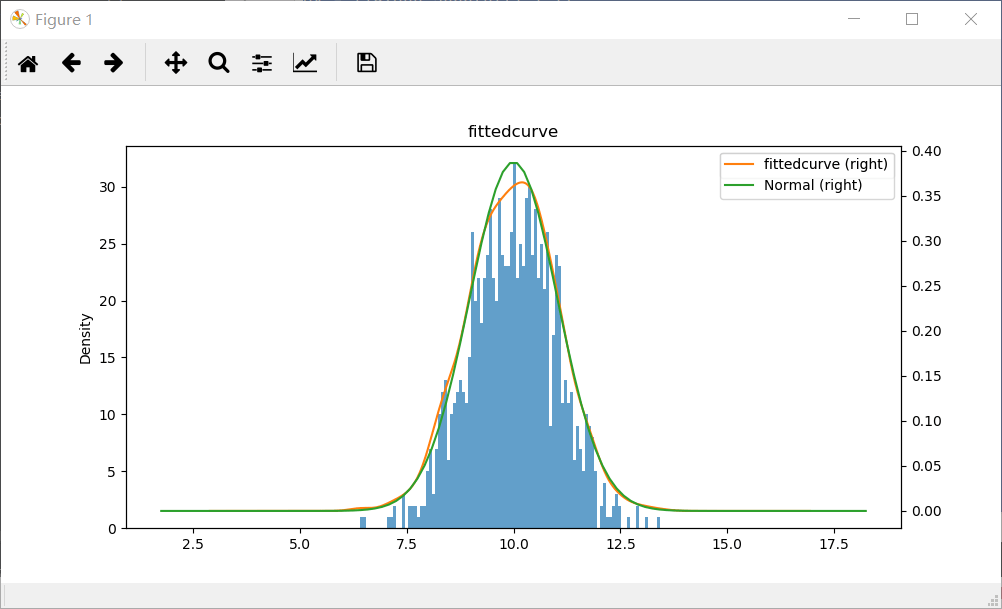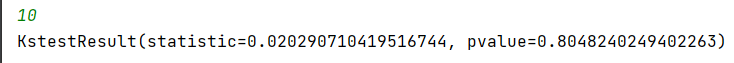# Python实现正态分布模型（Build Normal_distribution）

## 一、正态分布定义

正态分布（Normal distribution），也称“常态分布”，又名高斯分布（Gaussian distribution）是统计学中最重要的连续概率分布。

若随机变量X服从一个数学期望为μ、方差为σ2的正态分布，记为N(μ，σ2)。其概率密度函数为正态分布的期望值μ决定了其位置，其标准差σ决定了分布的幅度。当μ = 0,σ = 1时的正态分布是标准正态分布。

## 二、正态分布性质

1、曲线下的总面积为 1

2、随机变量 X 等于任何特定值的概率为 0 .

3、X 大于 a 的概率等于以 a 为界到正无穷大的曲线下的面积

4、X 小于 a 的概率等于从负无穷大到以 a 为界的曲线下的面积

集中性：正态曲线的高峰位于正中央，即均数所在的位置。

对称性：正态曲线以均数为中心，左右对称，曲线两端永远不与横轴相交。

均匀变动性：正态曲线由均数所在处开始，分别向左右两侧逐渐均匀下降。

## 四、正态分布试验设计及实现

import numpy as np
import pandas as pd
import matplotlib.pyplot as plt
from scipy import stats
K = input() # 设定均值
K = int(K) # 强制转换均值为整型
S = pd.DataFrame(np.random.randn(1000)+K,columns=['fittedcurve']) # np.random.randn(1000)+K为创造1000个均值为K的点，服从正态分布，np.random.poisson()为服从泊松分布
fig = plt.figure(figsize=(10,5)) # 设定画布
S.hist(bins=100,alpha=0.7,ax=ax)
S.plot(kind='kde',secondary_y=True,ax=ax)
std = S['fittedcurve'].std()
print(stats.kstest(S['fittedcurve'],'norm',(K,std)))
X = np.linspace(K-8*std,K+8*std,100)
def guass(data,avg,sig):
sqrt_2pi = np.power(2*np.pi,0.5)   # 对（2*π）进行开方
coef = 1/(sqrt_2pi*sig)   # 1/（（2*π）进行开方乘标准差）
powercoef = -1/(2*np.power(sig,2))    # -1/（2*sig*sig）
mypow = powercoef*(np.power((data-avg),2))   # mypow计算
return coef*(np.exp(mypow))    # cofe乘e的mypow次方
Y = []
for i in range(100):
S = guass(X[i],K,std)
Y.append(S)
plt.plot(X,Y,label='Normal')
plt.legend(['fittedcurve (right)','Normal (right)'],facecolor='white')
plt.show()